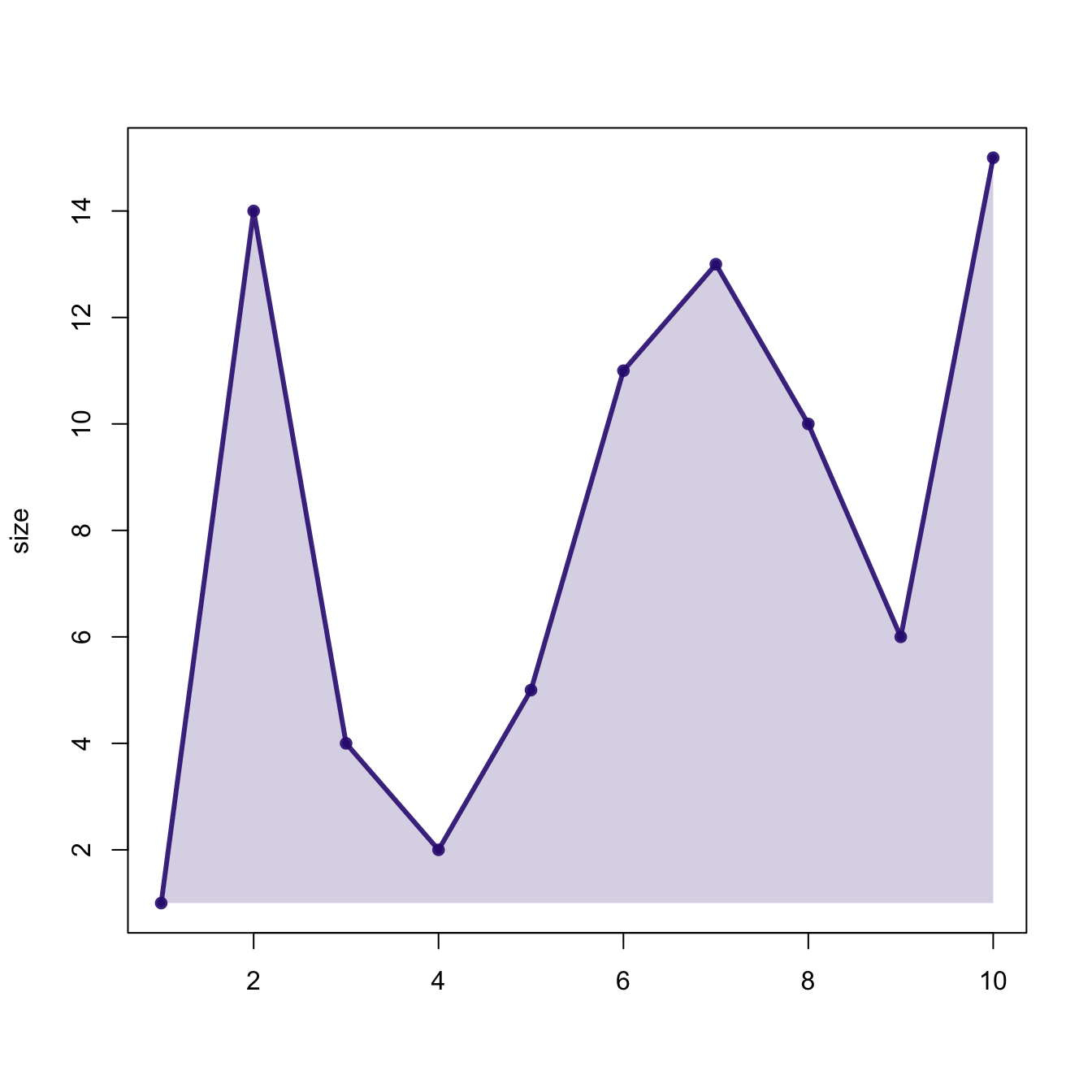# Area chart with base R

This post describes how to build an area chart using base R and the `polygon()` function. See the area chart section for a `ggplot2` implementation.

# Basic area chart with `plot()` and `polygon()`

Base R also allows to build area charts thanks to the `polygon()` function. This function requires 2 inputs: `x` and `y`.

Note that extreme values of both are added at the beginning and at the end of each vectors to make sure the polygon is closed.``````# Create data
data <- data.frame(x=seq(1,10), y=sample(seq(1,15),10))

# Draw line on top
plot(data, col=rgb(0.2,0.1,0.5,0.9), type="o", lwd=3, xlab="", ylab="size",
pch=20)

# Fill the area
polygon(c(min(data\$x), data\$x, max(data\$x)),
c(min(data\$y), data\$y, min(data\$y)),
col=rgb(0.2,0.1,0.5,0.2), border=FALSE)``````

Related chart types

## Contact

This document is a work by Yan Holtz. Any feedback is highly encouraged. You can fill an issue on Github, drop me a message on Twitter, or send an email pasting yan.holtz.data with gmail.com.